• +91 9971497814
• info@interviewmaterial.com

# RD Chapter 30- Derivatives Ex-30.4 Interview Questions Answers

### Related Subjects

Question 1 : Differentiate the following functions with respect to x:

x3 sin x

Let us consider y = x3 sinx

We need to find dy/dx

We know that y is a product of two functions say u and v where,

u = x3 and v =sin x

y= uv

Now let us apply product rule of differentiation.

By using product rule, we get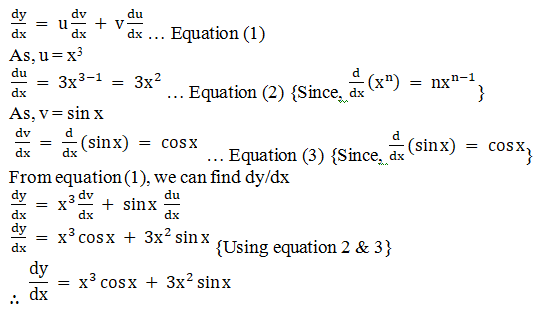Question 2 :

x3 ex

Let us consider y = x3 ex

We need to find dy/dx

We know that y is a product of two functions say u and v where,

u = x3 and v = ex

y= uv

Now let us apply product rule of differentiation.

By using product rule, we get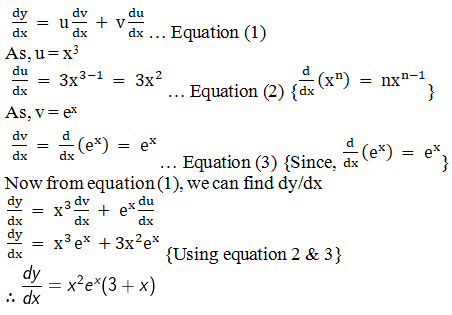Question 3 :

x2 ex logx

Let us consider y = x2 ex log x

We need to find dy/dx

We know that y is a product of two functions say u and v where,

u = x2 and v = ex

y= uv

Now let us apply product rule of differentiation.

By using product rule, we get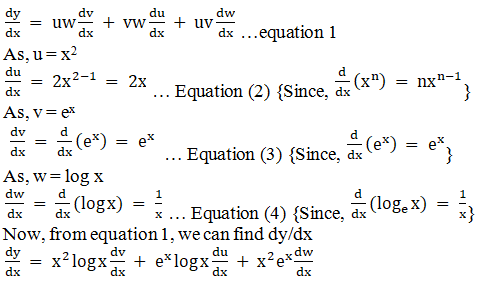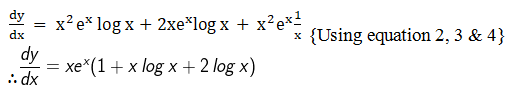Question 4 :

xn tan x

Let us consider y = xn tanx

We need to find dy/dx

We know that y is a product of two functions say u and v where,

u = xn and v =tan x

y= uv

Now let us apply product rule of differentiation.

By using product rule, we get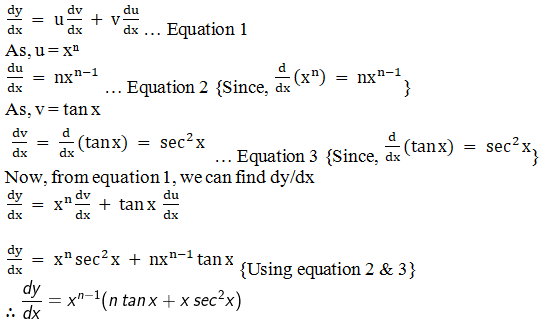Question 5 :

xn loga x

Let us consider y = xn loga x

We need to find dy/dx

We know that y is a product of two functions say u and v where,

u = xn and v = loga x

y= uv

Now let us apply product rule of differentiation.

By using product rule, we get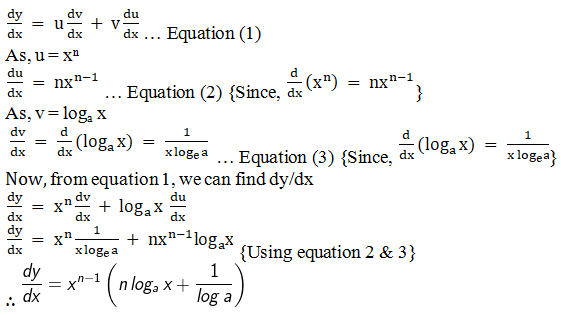Todays Deals### RD Chapter 30- Derivatives Ex-30.4 Contributorskrishan

Name:
Email:

# Latest News# 9000 interview questions in different categories